# RD Sharma Solutions for Class 6 Maths Chapter 5: Negative Numbers and Integers Exercise 5.2

## RD Sharma Solutions for Class 6 Maths Exercise 5.2 PDF

Mathematics is one of the challenging subjects which requires regular practice and conceptual knowledge. The students can improve their logical thinking and problem solving abilities by using the RD Sharma Solutions. The experts at BYJU’S solve the problems from the RD Sharma textbook of latest CBSE syllabus. The primary objective of creating solutions is to help students perform well in the final exam. The solutions created by the subject matter experts are accurate from the exam point of view. RD Sharma Solutions for Class 6 Maths Chapter 5 Negative Numbers and Integers Exercise 5.2 are provided here.

## RD Sharma Solutions for Class 6 Maths Chapter 5: Negative Numbers and Integers Exercise 5.2 Download PDF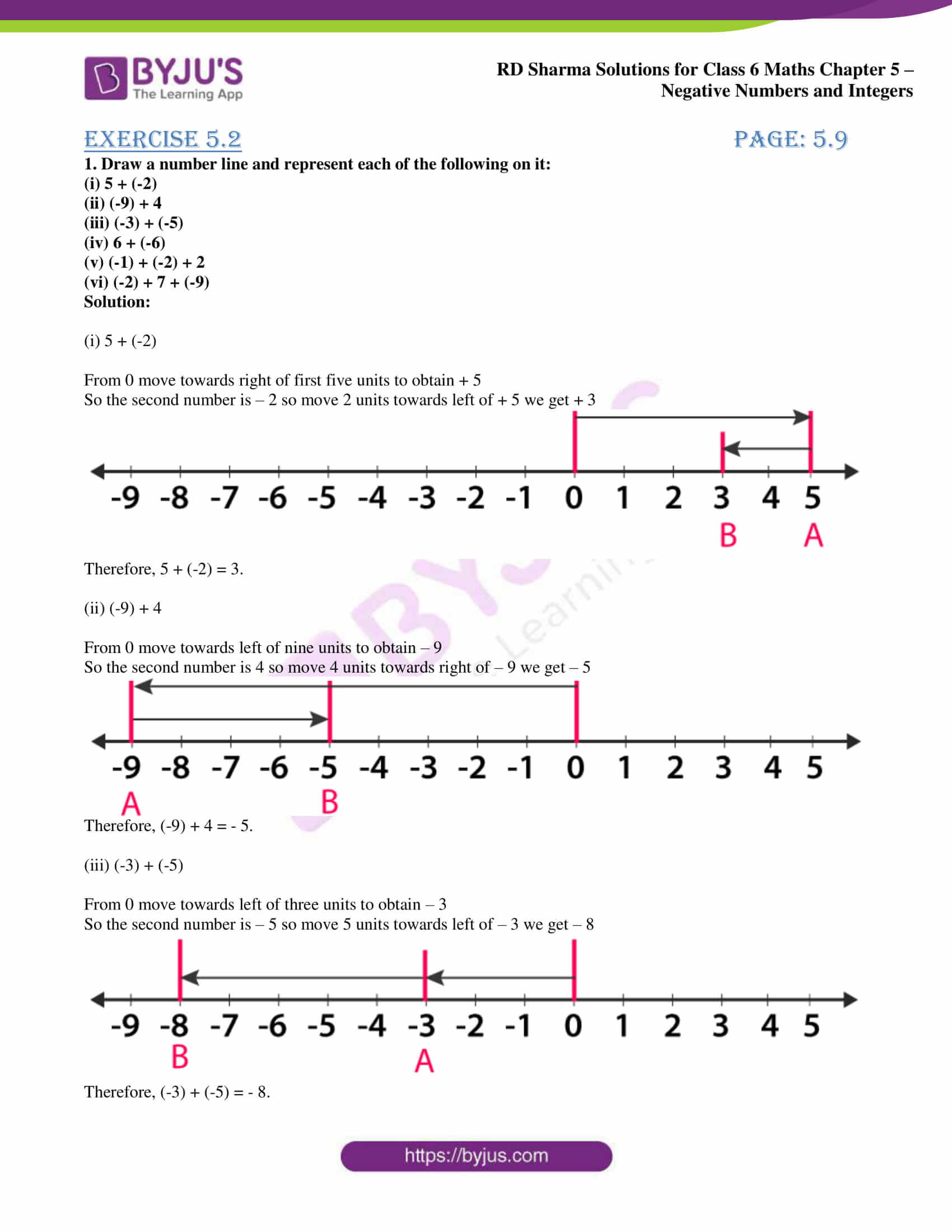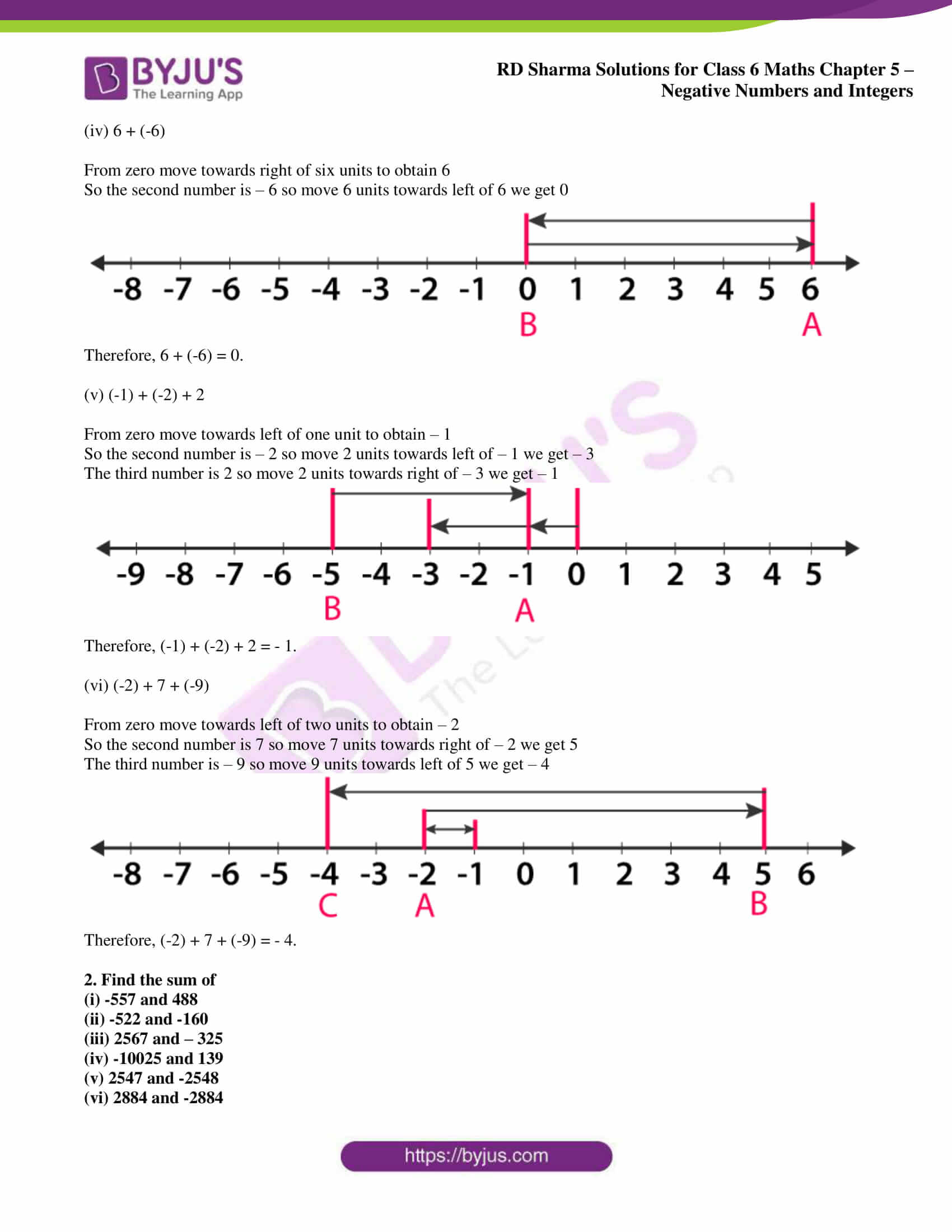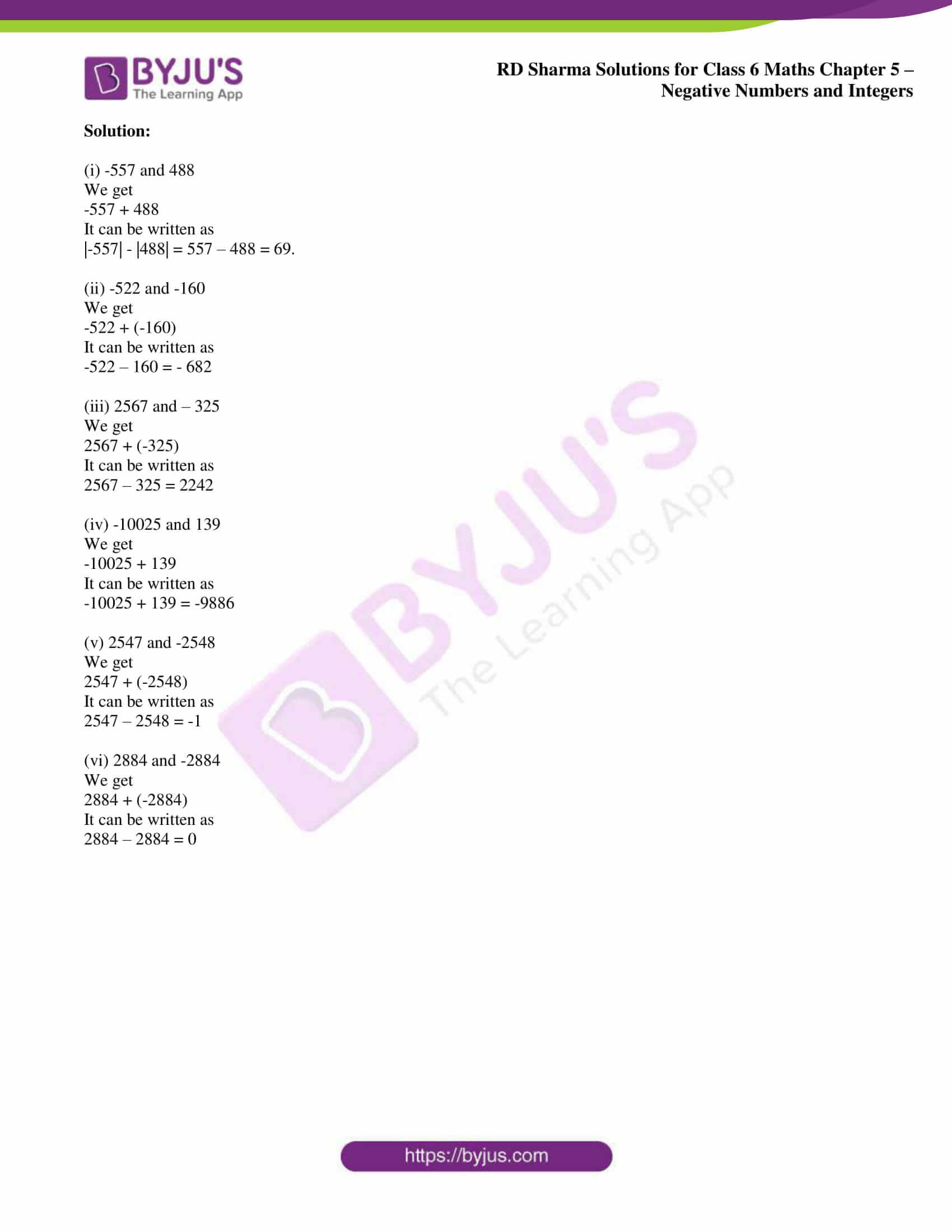## Access answers to Maths RD Sharma Solutions for Class 6 Chapter 5: Negative Numbers and Integers Exercise 5.2

1. Draw a number line and represent each of the following on it:

(i) 5 + (-2)

(ii) (-9) + 4

(iii) (-3) + (-5)

(iv) 6 + (-6)

(v) (-1) + (-2) + 2

(vi) (-2) + 7 + (-9)

Solution:

(i) 5 + (-2)

From 0 move towards right of first five units to obtain + 5

So the second number is – 2 so move 2 units towards left of + 5 we get + 3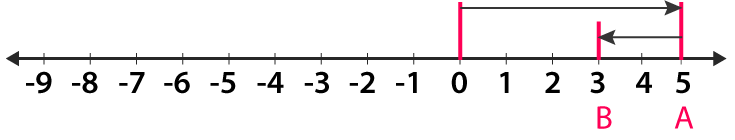Therefore, 5 + (-2) = 3.

(ii) (-9) + 4

From 0 move towards left of nine units to obtain – 9

So the second number is 4 so move 4 units towards right of – 9 we get – 5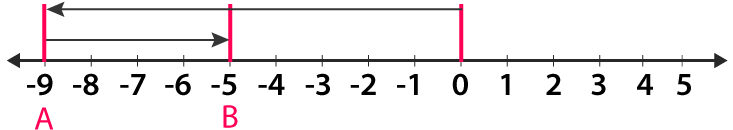Therefore, (-9) + 4 = – 5.

(iii) (-3) + (-5)

From 0 move towards left of three units to obtain – 3

So the second number is – 5 so move 5 units towards left of – 3 we get – 8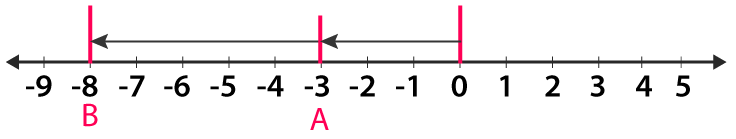Therefore, (-3) + (-5) = – 8.

(iv) 6 + (-6)

From zero move towards right of six units to obtain 6

So the second number is – 6 so move 6 units towards left of 6 we get 0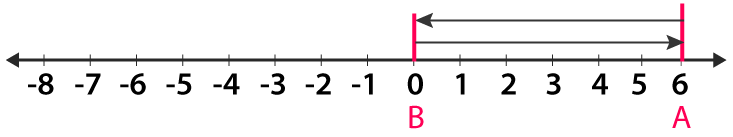Therefore, 6 + (-6) = 0.

(v) (-1) + (-2) + 2

From zero move towards left of one unit to obtain – 1

So the second number is – 2 so move 2 units towards left of – 1 we get – 3

The third number is 2 so move 2 units towards right of – 3 we get – 1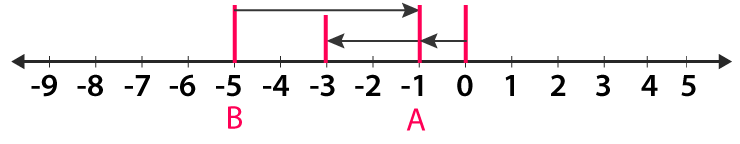Therefore, (-1) + (-2) + 2 = – 1.

(vi) (-2) + 7 + (-9)

From zero move towards left of two units to obtain – 2

So the second number is 7 so move 7 units towards right of – 2 we get 5

The third number is – 9 so move 9 units towards left of 5 we get – 4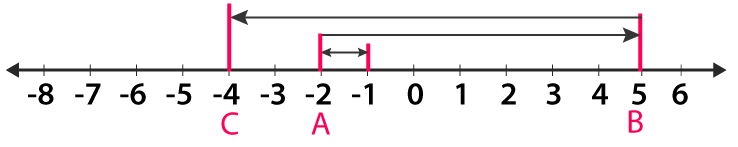Therefore, (-2) + 7 + (-9) = – 4.

2. Find the sum of

(i) -557 and 488

(ii) -522 and -160

(iii) 2567 and – 325

(iv) -10025 and 139

(v) 2547 and -2548

(vi) 2884 and -2884

Solution:

(i) -557 and 488

We get

-557 + 488

It can be written as

|-557| – |488| = 557 – 488 = 69.

(ii) -522 and -160

We get

-522 + (-160)

It can be written as

-522 – 160 = – 682

(iii) 2567 and – 325

We get

2567 + (-325)

It can be written as

2567 – 325 = 2242

(iv) -10025 and 139

We get

-10025 + 139

It can be written as

-10025 + 139 = -9886

(v) 2547 and -2548

We get

2547 + (-2548)

It can be written as

2547 – 2548 = -1

(vi) 2884 and -2884

We get

2884 + (-2884)

It can be written as

2884 – 2884 = 0

### RD Sharma Solutions for Class 6 Maths Chapter 5 – Negative Numbers and Integers Exercise 5.2

RD Sharma Solutions Class 6 Maths Chapter 5 Negative Numbers and Integers Exercise 5.2 consists of important concepts like addition of integers and procedure followed in solving problems.

### Key features of RD Sharma Solutions for Class 6 Maths Chapter 5: Negative Numbers and Integers Exercise 5.2

• RD Sharma Solutions help students to revise important concepts from the exam point of view.
• The students can self assess their knowledge about the concepts which are covered in each exercise.
• Solving a huge number of problems mainly improves efficiency in answering the questions based on the respective concepts.
• It helps students to know about the various types of problems that would appear in the exam.